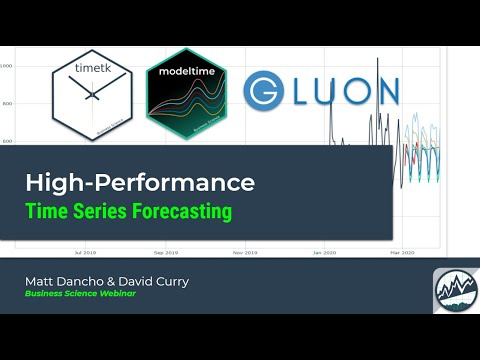# timetk## Mission

To make it easy to visualize, wrangle, and feature engineer time series data for forecasting and machine learning prediction.

## Installation

``remotes::install_github("business-science/timetk")``

``install.packages("timetk")``

## Package Functionality

There are many R packages for working with Time Series data. Here’s how `timetk` compares to the “tidy” time series R packages for data visualization, wrangling, and feature engineeering (those that leverage data frames or tibbles).

Structure
Data Structure tibble (tbl) tsibble (tbl_ts) tsibble (tbl_ts) tibbletime (tbl_time)
Visualization
Interactive Plots (plotly) :x: :x: :x:
Static Plots (ggplot) :x: :x:
Time Series :x: :x:
Correlation, Seasonality :x: :x:
Anomaly Detection :x: :x: :x:
Data Wrangling
Time-Based Summarization :x: :x:
Time-Based Filtering :x: :x:
Low to High Frequency :x: :x: :x:
Imputation :x: :x:
Sliding / Rolling :x:
Feature Engineering (recipes)
Date Feature Engineering :x: :x: :x:
Holiday Feature Engineering :x: :x: :x:
Fourier Series :x: :x: :x:
Smoothing & Rolling :x: :x: :x:
Imputation :x: :x: :x:
Cross Validation (rsample)
Time Series Cross Validation :x: :x: :x:
Time Series CV Plan Visualization :x: :x: :x:
More Awesomeness
Making Time Series (Intelligently) :x:
Handling Holidays & Weekends :x: :x: :x:
Class Conversion :x: :x:
Automatic Frequency & Trend :x: :x: :x:

## What can you do in 1 line of code?

Investigate a time series…

``````taylor_30_min %>%
plot_time_series(date, value, .color_var = week(date),
.interactive = FALSE, .color_lab = "Week")``````Visualize anomalies…

``````walmart_sales_weekly %>%
group_by(Store, Dept) %>%
plot_anomaly_diagnostics(Date, Weekly_Sales,
.facet_ncol = 3, .interactive = FALSE)``````Make a seasonality plot…

``````taylor_30_min %>%
plot_seasonal_diagnostics(date, value, .interactive = FALSE)``````Inspect autocorrelation, partial autocorrelation (and cross correlations too)…

``````taylor_30_min %>%
plot_acf_diagnostics(date, value, .lags = "1 week", .interactive = FALSE)``````## Acknowledgements

The `timetk` package wouldn’t be possible without other amazing time series packages.

• stats - Basically every `timetk` function that uses a period (frequency) argument owes it to `ts()`.
• `plot_acf_diagnostics()`: Leverages `stats::acf()`, `stats::pacf()` & `stats::ccf()`
• `plot_stl_diagnostics()`: Leverages `stats::stl()`
• lubridate: `timetk` makes heavy use of `floor_date()`, `ceiling_date()`, and `duration()` for “time-based phrases”.
• Add and Subtract Time (`%+time%` & `%-time%`): `"2012-01-01" %+time% "1 month 4 days"` uses `lubridate` to intelligently offset the day
• xts: Used to calculate periodicity and fast lag automation.
• forecast (retired): Possibly my favorite R package of all time. It’s based on `ts`, and it’s predecessor is the `tidyverts` (`fable`, `tsibble`, `feasts`, and `fabletools`).
• The `ts_impute_vec()` function for low-level vectorized imputation using STL + Linear Interpolation uses `na.interp()` under the hood.
• The `ts_clean_vec()` function for low-level vectorized imputation using STL + Linear Interpolation uses `tsclean()` under the hood.
• Box Cox transformation `auto_lambda()` uses `BoxCox.Lambda()`.
• tibbletime (retired): While `timetk` does not import `tibbletime`, it uses much of the innovative functionality to interpret time-based phrases:
• `tk_make_timeseries()` - Extends `seq.Date()` and `seq.POSIXt()` using a simple phase like “2012-02” to populate the entire time series from start to finish in February 2012.
• `filter_by_time()`, `between_time()` - Uses innovative endpoint detection from phrases like “2012”
• `slidify()` is basically `rollify()` using `slider` (see below).
• slider: A powerful R package that provides a `purrr`-syntax for complex rolling (sliding) calculations.
• `slidify()` uses `slider::pslide` under the hood.
• `slidify_vec()` uses `slider::slide_vec()` for simple vectorized rolls (slides).
• padr: Used for padding time series from low frequency to high frequency and filling in gaps.
• The `pad_by_time()` function is a wrapper for `padr::pad()`.
• See the `step_ts_pad()` to apply padding as a preprocessing recipe!
• TSstudio: This is the best interactive time series visualization tool out there. It leverages the `ts` system, which is the same system the `forecast` R package uses. A ton of inspiration for visuals came from using `TSstudio`.

## Learning MoreMy Talk on High-Performance Time Series Forecasting

Time series is changing. Businesses now need 10,000+ time series forecasts every day. This is what I call a High-Performance Time Series Forecasting System (HPTSF) - Accurate, Robust, and Scalable Forecasting.

High-Performance Forecasting Systems will save companies MILLIONS of dollars. Imagine what will happen to your career if you can provide your organization a “High-Performance Time Series Forecasting System” (HPTSF System).

I teach how to build a HPTFS System in my High-Performance Time Series Forecasting Course. If interested in learning Scalable High-Performance Forecasting Strategies then take my course. You will learn:

• Time Series Machine Learning (cutting-edge) with `Modeltime` - 30+ Models (Prophet, ARIMA, XGBoost, Random Forest, & many more)
• NEW - Deep Learning with `GluonTS` (Competition Winners)
• Time Series Preprocessing, Noise Reduction, & Anomaly Detection
• Feature engineering using lagged variables & external regressors
• Hyperparameter Tuning
• Time series cross-validation
• Ensembling Multiple Machine Learning & Univariate Modeling Techniques (Competition Winner)
• Scalable Forecasting - Forecast 1000+ time series in parallel
• and more.

Unlock the High-Performance Time Series Forecasting Course